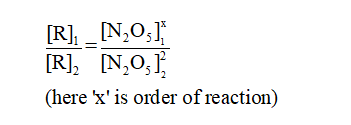Question
15 views

Determine the order of the decomposition reaction of N2O5, by using the initial rate data from the given table:

check_circle

Step 1

Chemical kinetics is the branch of chemistry that mainly deals with the rate of reaction and change in the concentration of reactant and product molecules with time.

Step 2

The rate of reaction expresses by the rate law which states that the rate of reaction is directly proportional to the active concentration of reactant molecules.

Step 3

Given:

[N2O5]  = 0.200 M then Rate = 0.0250 M/sec

[N2O5]  = 0.400 M then Rate = 0.400 M/sec...

### Want to see the full answer?

See Solution

#### Want to see this answer and more?

Solutions are written by subject experts who are available 24/7. Questions are typically answered within 1 hour.*

See Solution
*Response times may vary by subject and question.
Tagged in

### Chemistry